Physic Tutorials

How to solve questions on wave motion

How to solve questions on wave motion

Question 1

Given the progressive wave equation{UTME 2008}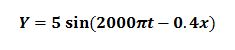calculate the wavelength.
A. 12.4m                            B. 15.7m                   C. 17.5m                                  D. 18.6m

Solution

using the wave equation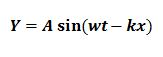compare this wave equation with the one given in the question

K is the phase difference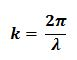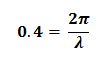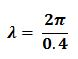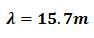B is the correct option

Question 2

If a light wave has a wavelength of 500nm in air, what is the frequency of the wave?{UTME 2009}
A. 3.0 x 1014 Hz                  B. 6.0 x 1014 Hz              C. 6.0 x 1012 Hz                   D. 2.5 x 1014 Hz
[c = 3 x 10^8 ms-1]

v = f

nanometer = 10^-9 m

3 x 10^8 = f x 500 x 10^-9

f = 3 x 10^8 / 500 x 10^-9

f = 6.0 x 10^14 Hz

B is the correct option

Question 3

The wavelength of a wave travelling with a velocity of 420ms-1 is 42 m. What is its
period?{UTME 2010}
A. 1.0s          B. 0.1s         C. 0.5s            D. 1.2s

Solution

v = / T

T = / v

T = 42 / 420

T = 0.1 s

B is the correct option

Question 4

A microphone connected to the Y-plates of a cathode-ray oscilloscope (c.r.o.) is placed in front of a loudspeaker. The trace on the screen of the c.r.o. is shown below{Cambridge may/june 2016 p12}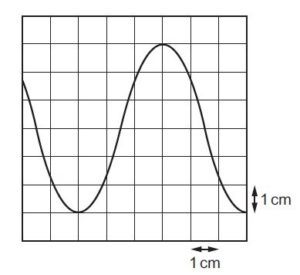The time-base setting is 0.5 ms cm–1 and the Y-plate sensitivity is 0.2 mV cm–1. What is the frequency of the sound from the loudspeaker and what is the amplitude of the trace on the c.r.o.?{ Cambridge may/june 2016 p12}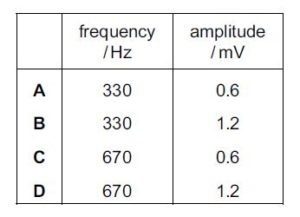Solution

The y-sensitivity will measure the amplitide which is on the y-axis

The time-base setting will measure the period which is on the x-axis

From the graph representation a full cycle occupies 6boxes

and one box to the x-axis o.5ms

period (T) = no of boxes x value of one box(which is 0.5 ms)

T = 6 X o.5 = 3 ms

Frequency(f) = 1 /T

f = 1 / (3 x 10^-3) = 333 Hz = 330 Hz approximately

from the graph the amplitude occupies 3 boxes i.e. maximum displacement from the rest position

and one box to the y-axis o.2 mv

A mplitude = no of boxes x no of one box(which is 0.2mv)

A = 3 x 0.2mv

A = 0.6 mv

A is the correct option

Question 5

The variation with time t of the displacement y of a wave X, as it passes a point P, is shown in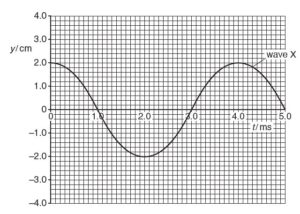use the diagram to determine the frequency of wave X.{ Cambridge may/june 2016 p12}

from the graph the period is 4.0 ms

f = 1 / T

f = 1 / (4 x 10^-3)

f = 250 Hz

Recommended: Click here to read short note on wave motion

Bolarinwa Olajire

A lecturer, Educationist, PhD student at FUNAAB, and a Blogger.